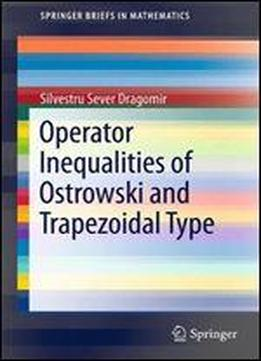# Operator Inequalities Of Ostrowski And Trapezoidal Type (springerbriefs In Mathematics) by Silvestru Sever Dragomir / 2011 / English / PDF

Inequalities of Ostrowski and Trapezoidal Type for Functions of Selfadjoint Operators on Hilbert Spaces presents recent results concerning Ostrowski and Trapezoidal type inequalities for continuous functions of bounded Selfadjoint operators on complex Hilbert spaces. The first chapter recalls some fundamental facts concerning bounded Selfadjoint operators on complex Hilbert spaces. The generalized Schwarz's inequality for positive Selfadjoint operators as well as some results for the spectrum of this class of operators are presented. The author also introduces and explores the fundamental results for polynomials in a linear operator, continuous functions of selfadjoint operators that will play a central role throughout the book. The following chapter is devoted to the Ostrowski's type inequalities, which provide sharp error estimates in approximating the value of a function by its integral mean and can be used to obtain a priory error bounds for different quadrature rules in approximating the Riemann integral by different Riemann sums. The author also presents recent results extending Ostrowski inequality in various directions for continuous functions of selfadjoint operators in complex Hilbert spaces. The final chapter illustrates recent results obtained in extending trapezoidal type inequality in various directions for continuous functions of selfadjoint operators in complex Hilbert spaces. Applications for mid-point inequalities and some elementary functions of operators as also provided. This book is intended for use by researchers in various fields of Linear Operator Theory and Mathematical Inequalities. As well as postgraduate students and scientists applying inequalities in their specific areas.

views: 173